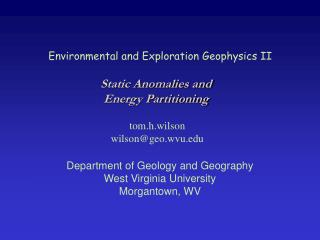Download PresentationEnvironmental and Exploration Geophysics II

# Environmental and Exploration Geophysics II - PowerPoint PPT PresentationDownload Presentation## Environmental and Exploration Geophysics II

- - - - - - - - - - - - - - - - - - - - - - - - - - - E N D - - - - - - - - - - - - - - - - - - - - - - - - - - -
##### Presentation Transcript

1. Environmental and Exploration Geophysics II Static Anomalies and Energy Partitioning tom.h.wilson wilson@geo.wvu.edu Department of Geology and Geography West Virginia University Morgantown, WV

2. Original due dates - and pb 4.8 Due Today, Oct. 24th Due Tuesday, Oct. 29th Velocity Analysis Problem

3. Recall Energy partitioning

4. Geophone output is often designed to be proportional to pressure, particle velocity, acceleration or displacement. Land geophone output is typically proportional to particle velocity, while marine geophones record pressure variations.

5. V Interval Velocity Particle Velocity v

6. Pi PR PT Normal Incidence Raypaths Boundary Conditions

7. We can rewrite boundary condition 1 as The subscript P indicates that pressure variations are being considered in this case

8. From the wave equation, we have that This allows us to rewrite boundary condition II in terms of the pressures, as - By convention, up is negative, thus

9. Our two boundary conditions become which implies As a matrix equation, we have

10. Part II Geophone output is often designed to be proportional to pressure, particle velocity, acceleration or displacement. Land geophone output is typically proportional to particle velocity, while marine geophones record pressure variations.

11. V Interval Velocity Particle Velocity v

12. Note that Pv =Vv2 Thus Ev2

13. We have, as expected, a decrease of energy across the interface. Energy is conserved!

14. Compute and plot two-way interval transit times, two-way total reflection time, layer impedance and boundary reflection coefficients

15. Density, velocity and impedance plots are usually represented in step-plot form. The values as listed are constant through an interval and marked by abrupt discontinuity across layer boundaries.

16. Reflection coefficients exist only at boundaries across which velocity and density change, hence their value is everywhere 0 except at these boundaries. Stick Plot

17. Subsurface model Simplified representation of the source disturbance

18. Follow the wavefront through the subsurface and consider how its amplitude changes as a function only of energy partitioning. A. What is the amplitude of the disturbance at point A? B. At point B we have transmission through the interface separating media 1 and 2. At C? We consider only transmission and reflection losses. Geometrical divergence and absorption losses are ignored. Hence PA = 1psi. - hence the amplitude of the wavefront at B is Tp 12 PA.

19. At C? - At D? -

20. Consider for a moment- the general n-layer case.